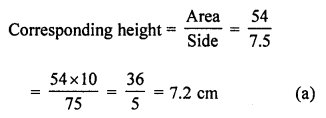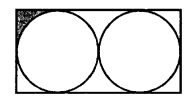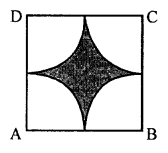## ML Aggarwal Class 7 Solutions for ICSE Maths Chapter 16 Perimeter and Area Objective Type Questions

Mental Maths

Question 1.
Fill in the blanks:
(i) The perimeter of a regular polygon = ……….. × length of a side.
(ii) The unit of measurement of the area is ……….
(iii) The perimeter of a rhombus is = 4 × ………
(iv) An area of 1 km2 = ……… hectare
(v) If the perimeter of a parallelogram is 40 cm and the length of one side is 12 cm, then the length of the adjacent side is ……
(vi) To find the cost of polishing a table-top, we need to find the ………. of the table-top.
(vii) The ratio of circumference to the diameter of a circle is ………..
(viii) If the area of a triangular piece of cardboard is 90 cm2, then the length of the altitude corresponding to 20 cm long base is ………. cm.
Solution:
(i) The perimeter of a regular polygon = Number of sides × length of a side.
(ii) The unit of measurement of the area is a square unit.
(iii) The perimeter of a rhombus is = 4 × length of side.
(iv) An area of 1 km2 = 1000 hectare
(v) If the perimeter of a parallelogram is 40 cm and the length of one side is 12 cm,
then the length of the adjacent side is 8 cm.
(vi) To find the cost of polishing a table-top, we need to find the area of the table-top.
(vii) The ratio of circumference to the diameter of a circle is π.
(viii) If the area of a triangular piece of cardboard is 90 cm2,
then the length of the altitude corresponding to 20 cm long base is 9 cm.

Question 2.
State whether the following statements are true (T) or false (F):
(i) A diagonal of a rectangle divides it into two right-angled triangles of equal areas.
(ii) A diagonal of a parallelogram divides it into two triangles of equal areas.
(iii) If the perimeter of two parallelograms is equal, then their areas are also equal.
(iv) All parallelogram having equal areas have the same perimeters.
(v) The area of a circle of diameter d is πd2.
(vi) Area of a parallelogram = product of lengths of its two adjacent sides.
Solution:
(i) A diagonal of a rectangle divides it into two
right-angled triangles of equal areas. (True)
(ii) A diagonal of a parallelogram divides it into
two triangles of equal areas. (True)
(iii) If the perimeter of two parallelograms is equal,
then their areas are also equal. (False)
(iv) All parallelogram having equal areas
have the same perimeters. (False)
(v) The area of a circle of diameter d is πd2. (False)
Correct:
Area of circle = πr2 (r is a radius of the circle).
(vi) Area of a parallelogram = product of lengths of its
Correct:
Area = Base × Corresponding altitude.

Multiple Choice Questions

Choose the correct answer from the given four options (3 to 14):
Question 1.
If the perimeter of a square is 24 cm, then its area is
(a) 16 cm2
(b) 24 cm2
(c) 36 cm2
(d) 36 m2
Solution:
Perimeter of a square = 24 cm
Side = $$\frac { 24 }{ 4 }$$ = 6 cm
Area = (Side)2 = 6 × 6 = 36 cm2 (c)

Question 4.
If the area of a parallelogram is 54 cm2 and the length of one side is 7.5 cm, then the corresponding height is
(a) 7.2 cm
(b) 14.4 cm
(c) 3.6 cm
(d) 13.5 cm
Solution:
Area of a ||gm = 54 cm2
Length of one side = 7.5 cmQuestion 5.
If the base of a triangle is doubled and its height is halved, then the area of the resulting triangle
(a) decreases
(b) increases
(c) doubles
(d) remains the same
Solution:
If the base is doubled and height is halved,
then the area of the resulting triangle remains the same. (d)

Question 6.
If the height of a parallelogram is doubled and base tripled, then its area becomes
(a) 2 times
(b) 3 times
(c) 6 times
(d) 12 times
Solution:
If the height of a ||gm is doubled and base tripled
then the area becomes 2 × 3 = 6 times (c)

Question 7.
The circumference of the circle with diameter 28 cm is
(a) 44 cm
(b) 88 cm
(c) 176 cm
(d) 616 cm
Solution:
The circumference of a circle with diameter
28 cm is = 28 × π = 28 × $$\frac { 22 }{ 7 }$$ = 88 cm (b)

Question 8.
The ratio of circumference to the area of a circle of radius r units is
(a) 2 : r
(b) r : 2
(c) 1 : r
(d) π : r
Solution:
The ratio of circumference to
the area of a circle of radius r units is
2πr : πr2 = 2 : r (a)

Question 9.
If the area of a circle is numerically equal to its circumference, then the radius of the circle is
(a) 1 unit
(b) 2 units
(c) 3 units
(d) 4 units
Solution:
Area of a circle is numerically equal to its
circumference the radius of the circle
πr2 = 2πr ⇒ r = 2 units (b)

Question 10.
The area of a circle of diameter d is
(a) 2πd2
(b) πd2
(c) $$\frac { \pi { d }^{ 2 } }{ 2 }$$
(d) $$\frac { \pi { d }^{ 2 } }{ 4 }$$
Solution:
The area of a circle of diameter d is
πr2 = $$\pi \left( \frac { d }{ 2 } \right) ^{ 2 }$$ = $$\frac { \pi { d }^{ 2 } }{ 4 }$$ (d)

Question 11.
If the ratio of the radii of two circles is 2 then the ratio of their circumferences is
(a) 2 : 3
(b) 3 : 2
(c) 4 : 9
(d) 9 : 4
Solution:
Let radii be 2x and 3x
Then ratio between their
Circumferences = 2πr1 : 2πr2
= 2π(2x) : 2π(3x)
= 2x : 3x
= 2 : 3 (a)

Question 12.
If the ratio of the radii of two circles is 3 : 5, then the ratio of their areas is
(a) 3 : 5
(b) 5 : 3
(c) 25 : 9
(d) 9 : 25
Solution:
The ratio of radii of two circles is 3 : 5
Let radii of two circles be 3x, and 5x
Then ratio between then areas be
π(3x)2 : π(5x)2 = 97πx2 : 25πx2
= 9 : 25 (d)

Question 13.
The perimeter of a semicircle (including its diameter) of radius 7 cm is
(a) 22 cm
(b) 29 cm
(c) 36 cm
(d) 44 cm
Solution:
The perimeter of a semicircle
(including diameter) of radius 7 cmQuestion 14.
Area of a rectangle and the area of a circle are equal. If the dimensions of the rectangle are 14 cm × 11 cm, then the radius of the circle is
(a) 21 cm
(b) 14 cm
(c) 10.5 cm
(d) 7 cm
Solution:
Area of a rectangle = Area of a circle
Dimensions of the rectangle are 14 cm × 11 cm
Area of circle = 14 × 11 = 154 cm2Higher Order Thinking Skills (HOTS)

Question 1.
In the given figure, ABDE is a parallelogram, find the area of the trapezium ACDE.Solution:
In the given figure,
ABDE is a parallelogram and ACDE is a trapezium
whose bases are 13 m and height = 6.5 m
Area = $$\frac { 1 }{ 2 }$$ (Sum of parallel sides) × Height
= $$\frac { 1 }{ 2 }$$ (13 + 7) × 6.5 m2
= $$\frac { 1 }{ 2 }$$ × 20 × 6.5 = 65 m2

Question 2.
In the given figure, the length of the rectangle is 28 cm. Find the area of the shaded region.Solution:
In the given figure,
Length of rectangle = 28 cm
Then diameter of each circle = $$\frac { 28 }{ 2 }$$ = 14 cm
and radius = $$\frac { 14 }{ 2 }$$ = 7 cm
Breadth of rectangle = 14 cmQuestion 3.
In the given figure, ABCD is a square of side 14 cm. A, B, C, and D are centers of circular arcs of equal radius. Find the perimeter and the area of the shaded region.Solution:
In the given figure, ABCD is a square of side 14 cm
Area of square = (Side)2 = (14)2 = 196 cm2
Now radius of each quadrant = $$\frac { 14 }{ 2 }$$ = 7 cm
Area of 4 quadrants = 7 × $$\frac { 1 }{ 4 }$$ × πr2
= $$\frac { 22 }{ 7 }$$ × 7 × 7 = 154 cm2
Area of shaded portion = Area of square – Area of 4 quadrant
= 196 – 154 = 42 cm2
and perimeter = 4 × $$\frac { 1 }{ 4 }$$ × 2πr
= 2πr = 2 × $$\frac { 22 }{ 7 }$$ × 7 = 44 cm